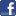# Roundoff Error

Robert P. Munafo, 1996 Dec 3.

There are two ways to look at roundoff error, which are essentially "pure" and "applied".

The pure approach looks at the theoretical error term in each calculation in the iteration of a point, to see how far a point can be iterated while still guaranteeing that the answer is accurate.

Iteration involves multiplication and addition (Z = Z2 + C = Z*Z + C) and the multiplication is the main source of the growth of the error term. A good rough estimate is that the error doubles with each iteration. This means that if our numbers have B binary digits of precision then after N iterations only the first B-N binary digits are accurate. So, an accurate picture of the Mandelbrot Set with a Dwell Limit of D and a grid spacing (distance between adjacent pixels) of 2-N can be accurately drawn using N+D bits of accuracy in the math.

Since most Mandelbrot views use dwell limits like 1000 or 10,000 and most floating point math libraries only support about 50 bits of accuracy, there seems to be a problem. What are the pictures we're looking at — are they actually related in any way to the actual appearance of the Mandelbrot Set?

In fact, they are. Experiments show that even when you write a 1000-bit floating point library, the pictures look the same as they did with 50-bit floating point. In fact a few pixels differ here and there, but there are no noticable differences even when you look very close. The fundamental reason for this is that the floating point roundoff is effectively insignificant compared to the non-linear mapping induced by the (chatotic) iteration itself. Unless an iterate Zn happens to fall on or near a critical point, the mapping actually serves to diminish the errors from previous steps and so the total error ends up being only about twice the roundoff from a single iteration.

The applied approach recognizes this and uses experimental methods to show how much error is introduced by roundoff. The primary interest is to generate views which are accurate in appearance, i.e. indistinguishable from a "perfect" version. Sometimes (e.g. when applying the Automatic Math-Precision algorithm) it is desirable to know how many bits you need for given coordinates (center and size).

The following was posted to sci.fractals in 1993 as part of the then-ongoing pixel-counting effort.

FLOATING-POINT ROUNDOFF: Here are the floating-point accuracy results:   width height max_iter pix_in_set area mantissa type 8192 4096 524288 8987154 1.50658906 24 float 8192 4096 524288 8987091 1.50657853 33 double, truncated 8192 4096 524288 8987089 1.50657820 44 double, truncated 8192 4096 524288 8987086 1.50657769 53 double 8192 4096 524288 8987088 1.50657803 64 extended   The "mantissa" column shows the number of mantissa bits, and "type" shows what data type was used in the computation. For the "double, truncated" types the following formula was applied to all intermediate results to simulate lowered resolution: truncated_result = (1001.0 * result) - (1000.0 * result) This example truncates about 10 bits; it reduces "float" to 14 bits and "double" to 44.   53-bit doubles are fairly common, and are often the highest precision available. 64-bit "extended" is the precision used in IEEE standard floating-point. On Intel and Motorola machines, it is the fastest precision available because it is the "native" precision for the hardware.   The pixel count seems to decrease as precision increases, approaching a limit around 8987087. The higher count for low precision is probably the result of roundoff error on a few points that are near the boundary of the Mandelbrot Set but not actually in the Set itself. Iterating these points, the roundoff error can result in a cycle (of very long period, probably); without roundoff error the iterations would not repeat and would eventually diverge.   It appears that the pixel count never really settles down on a specific value, but varies by one or two pixels depending on specifics of the floating-point precision used. This is not surprising as the 44-bit results were simulated using a roundoff formula that does not perfectly mimic what hardware would do, and the 64-bit results were done on a different machine from the other results.   Therefore, I think it is reasonable to put the error due to floating-point roundoff at 3 pixels on an 8192x4096 grid, which comes out to 0.00000050.

From the Mandelbrot Set Glossary and Encyclopedia, by Robert Munafo, (c) 1987-2018.     Mu-ency index

mrob27This page was written in the "embarrassingly readable" markup language RHTF, and was last updated on 2018 Feb 04.s.11